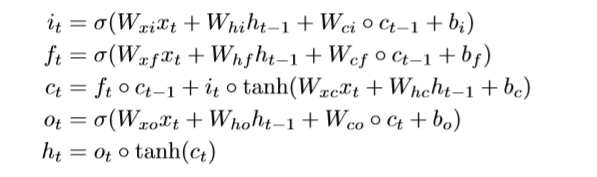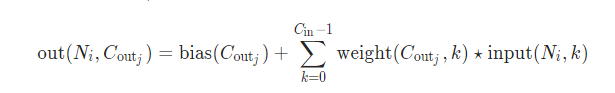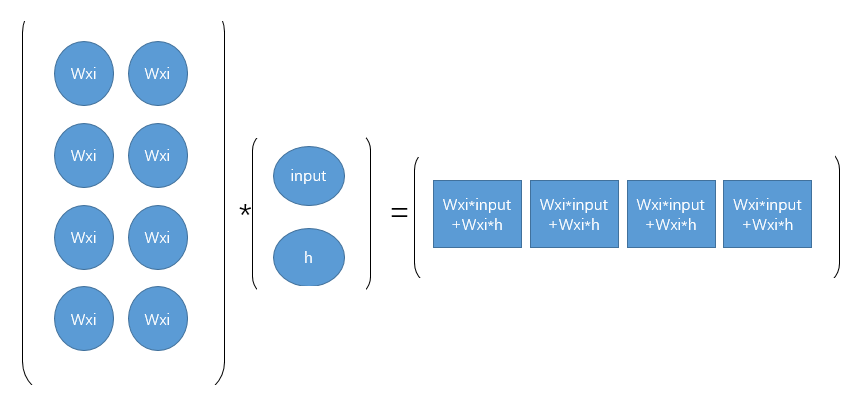## convLSTM 理解与实现_convlstm实现_Runner_of_nku的博客-程序员秘密``````class ConvLSTMCell(nn.Module):

def __init__(self, input_size, input_dim, hidden_dim, kernel_size, bias):
"""
Initialize ConvLSTM cell.

Parameters
----------
input_size: (int, int)
Height and width of input tensor as (height, width).
input_dim: int
Number of channels of input tensor.
hidden_dim: int
Number of channels of hidden state.
kernel_size: (int, int)
Size of the convolutional kernel.
bias: bool
Whether or not to add the bias.
"""

super(ConvLSTMCell, self).__init__()

self.height, self.width = input_size
self.input_dim = input_dim
self.hidden_dim = hidden_dim

self.kernel_size = kernel_size
self.padding = kernel_size // 2, kernel_size // 2
self.bias = bias

self.conv = nn.Conv2d(in_channels=self.input_dim + self.hidden_dim,
out_channels=4 * self.hidden_dim,
kernel_size=self.kernel_size,
bias=self.bias)

def forward(self, input_tensor, cur_state):
'''
:param input_tensor:[batch,dim,inp_height,inp_width]
:param cur_state: [h,c] h:[batch,dim,H,W]
:return:
'''
h_cur, c_cur = cur_state

combined = torch.cat([input_tensor, h_cur], dim=1)

combined_conv = self.conv(combined)
cc_i, cc_f, cc_o, cc_g = torch.split(combined_conv, self.hidden_dim, dim=1)
i = torch.sigmoid(cc_i)
f = torch.sigmoid(cc_f)
o = torch.sigmoid(cc_o)
g = torch.tanh(cc_g)

c_next = f * c_cur + i * g
h_next = o * torch.tanh(c_next)

return h_next, c_next

def init_hidden(self, batch_size):
return (Variable(torch.zeros(batch_size, self.hidden_dim, self.height, self.width)),
Variable(torch.zeros(batch_size, self.hidden_dim, self.height, self.width)))``````

cell的实现主要如上，其中可以看出来，参数是通过一次Conv2d的调用就申请好的，这里是这样理解的：

in_channels=self.input_dim + self.hidden_dim

out_channels=4 * self.hidden_dim这个公式可以理解为矩阵乘运算，不过矩阵乘运算中进行的是每个元素相乘相加，这里的矩阵乘运算是矩阵中每个核和每个输入的tensor进行卷积操作再相加。### sqlserver 字符串拆分和取某分隔符之前的字符串_weixin_33770878的博客-程序员秘密

ALTER FUNCTION [dbo].[f_splitSTR]( @s varchar(8000), --待分拆的字符串 @split varchar(10) --数据分隔符 )RETURNS @re TABLE( col varchar(max)) AS BEGIN DECLARE ...

### MATLAB 条形图或饼状图 图案填充_aizhao3648的博客-程序员秘密

function [im_hatch,colorlist] = applyhatch_pluscolor(h,patterns,CvBW,Hinvert,colorlist, ... dpi,hatchsc,lw)%APPLYHATCH_PLUSCOLOR Apply hatched...

### Mellanox Infiniband 架构设计快速实战指南 - A_ShawnTheLearner的博客-程序员秘密

Nvidia GTC 2020正在进行中，Nvidia（英伟达）最终完成了对以色列高速计算网络厂商Mellanox的收购，再一次被黄老板高度评价。Mellanox Infiniband至今依然是高速计算互联网络的主流厂商和解决方案，在HPC领域应用广泛。本文基于Mellanox官方提供的材料，介绍如何快速上手Infiniband高速互联网络的设计规划。